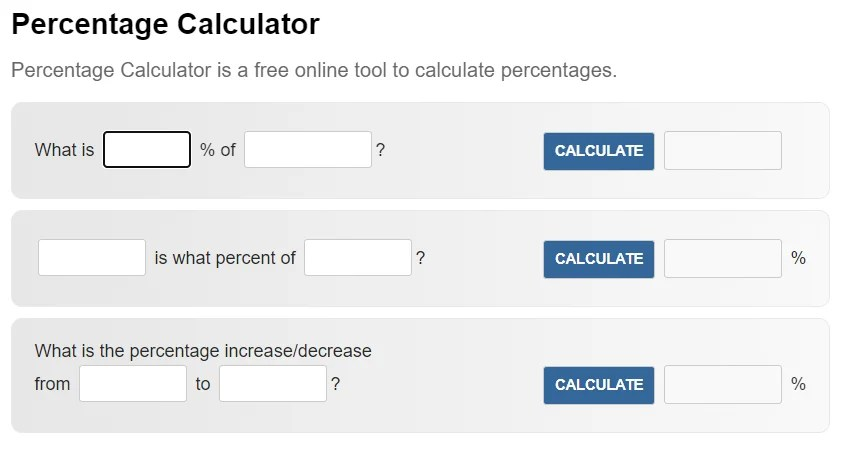# Percentage Calculator

Welcome to our website designed to make percentage calculations quick and easy for a variety of purposes. Whether you need to calculate discounts, tips or price changes, our percentage calculator is here to help.

## What is the percentage?

A percentage is a way of representing a number as a fraction of 100. It is usually used to express a part of a whole or to compare two numbers. Percentages are often represented with the symbol “%”. For example, if there are 100 cars in a garage and 25 of them are white, we can say that 25% of the cars in the garage are white.

## How do you calculate percentages?

To calculate a percentage, you usually divide the part (the smaller value) by the whole (the larger value) and then multiply the result by 100. This gives you the percentage value as a number between 0 and 100. For example, if you have 50 apples and you want to know what percent of them are red, and 20 of them are red, you would divide 20 by 50 to get 0.4, then get 40%. To do this, multiply by 100.

## Why do percentages matter?

Percentages play an important role in a variety of contexts, from calculating exemptions and taxes to measuring changes in stock prices and economic indicators. Understanding how percentages work empowers you to make informed decisions in personal finance and business management, among other areas.

## Percentage Calculator: Simplify Your Percentage Calculations

Introduction: Welcome to our Percentage Calculator, a user-friendly tool designed to simplify various percentage calculations for a wide range of purposes. Whether you need to determine discounts, tips, or percentage changes, our calculator is here to assist you effortlessly.

### Key Features:

1. Easy-to-Use Interface: Our Percentage Calculator features a straightforward interface, making it accessible to all users, regardless of their mathematical background.
2. Versatile Functions: From calculating discounts during sales to determining tip amounts at restaurants, our calculator caters to diverse percentage-related scenarios.
3. Precise Results: The calculator ensures accurate results, enabling you to make informed decisions with confidence.
4. Swift Calculations: Say goodbye to manual percentage computations; our calculator delivers quick results, saving you time and effort.
5. Mobile-Friendly: Our calculator is designed to work seamlessly on various devices, making it convenient to use anytime, anywhere.

## Popular Percentage Calculations:

• Calculate Discounts: Easily find the discounted price of an item during a sale or promotion.
• Determine Tips: Quickly calculate the appropriate tip amount for exceptional service at restaurants or other services.
• Percentage Increase or Decrease: Discover the percentage change between two values, such as stock prices or financial indicators.

## Conclusion:

Our Percentage Calculator is your go-to tool for all your percentage-related calculations. Whether you’re an individual managing personal finances or a business professional analyzing financial data, our calculator streamlines percentage computations with ease. Embrace the efficiency and accuracy of our tool, and simplify your percentage calculations today.